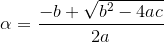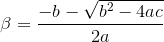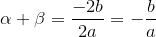Search IntMath
Close

450+ Math Lessons written by Math Professors and Teachers

5 Million+ Students Helped Each Year

1200+ Articles Written by Math Educators and Enthusiasts

Simplifying and Teaching Math for Over 23 Years

# How to search for solutions to math questions

By Murray Bourne, 24 Apr 2019

I recently updated the search facility on IntMath (so it no longer contains advertisements for other sites). I've been monitoring the searches to make sure all is working well.

It concerns me a lot of searches made by readers produce poor results. In many cases the topic does exist on IntMath, but the search engine couldn't find what the student was looking for, often because of the way the search was entered. (Real examples of such searches are used in this article.)

Jakob Nielsen, user experience expert, wrote College Students on the Web (2010) and Incompetent Research Skills Curb Users' Problem Solving (2011), bemoaning the state of students' search skills. While search engines have improved a lot, search skills still have a long way to go.

So here are some hints about how to be more successful when searching for an answer to a math question.Many search are very specific to a particular question, and so result in few results.

Find the title of the chapter or section in your text book (or lecture notes) and begin your search with that.

For example, these searches work well, because they are general enough and contain topic titles typically used in math books:

You'll notice in each case there is a useful page in the first two or three links given in the search result.

## Spend time with the search result

A lot of students spend just a few seconds looking at the page, hoping to find their exact question there, and so miss the key information provided - information that could help them to solve their problem.

Yes, we all want a "quick fix", but it's far better to grasp the big picture (and learn key concepts) than it is to waste a lot of time trying to find something which may not be there.

### The answer you want may be hidden on the page

A lot of sites, IntMath included, don't show all the text of a page when it first loads. IntMath includes many Examples and Exercises on each page, and the answers are not immediately visible. Clicking on the "Answer" link makes each answer visible. When users spend just a few seconds looking at a page, I know they can't be discovering the value of those "Answer" sections.

## Include the context

There are situations in math where the same word has different meanings.

For example, integral can mean:

• The adjective from the word "integer" (the numbers ... −3, −2, −1, 0, 1, 2, 3 ...); OR
• The result of performing an integration (the opposite process to differentiation) in calculus

You'll find both meanings given in this search result:

To get the one you actually want, add the context, like one of these:

## Use correct spelling!

While Google is very forgiving of spelling errors, you are more likely to find what you really want if you enter the correct spelling in your search query.

You'll need to know the correct spelling for when you communicate your answers later, so it's a good idea to learn the correct spelling early!## Use math language carefully

The language used in mathematics is for the most part very precise. "Solve" is not the same as "find the value of".

• "Solve" suggests we need to find the value of a variable (often x, for example "Solve x + 7 = 10"), while
• "Find the value of" means we need to perform some calculations to arrive at a final value of an expression (e.g. find the area of the circle with radius 3 cm").

The following search will not end well, because it is not precisely expressed:

The question might mean:

• Simplify the right side (which would give us y = 5x)
• Solve for x (which would be x = y/5)
• Graph the line y = 5x (which is a straight line, slope 5, passing through the origin)

Search engines look at user intent and if they can't work out what you want, they can't give good responses.

Actually, I suspect the use really wanted to search for

If so, the original question was probably:

## Tips for answering a specific math question

One of the search queries was as follows:

As mentioned above, this will rarely result in a useful search result. Sometimes, a really long query gives you "no results found".

In this case, the wording of the question is quite confused. I think it was supposed to be:

Find the quadratic equation with roots alpha and beta given alpha minus beta equals two and alpha squared minus beta squared equals three.

Using algebra, this means:

Find the quadratic equation with roots α and β given αβ = 2 and α2β2 = 3

Let's go through how we could approach this problem.

### i. First, remind yourself of key concepts

If the question comes from a text book (I hope it was better expressed if it did), go back and read over what a quadratic equation is, and how to find its roots (where it will equal zero, i.e. cut the x-axis).

If you wanted to use search (rather than read your text book, or lesson notes), these two searches would be a good place to start:

You would need to spend some time to read over the pages you find and to try out the examples.

### ii. What are those "alpha" and "beta" about?

The question says the roots α and β and we know from what we've just read that the roots are given by:and### iii. But the question talks about alpha squared and beta squared?

We will need to do some manipulation to get the roots into the format we need.

It's unlikely any site will have exactly what is needed for this question, but after following through several examples from several sites, hopefully you would gain enough insight.

I added a page to IntMath on this topic, and included this question as Example 2. See Sum and product of the roots of a quadratic equation

## Avoid the following

### a. Avoid any reference to grade level

This applies also to words like "class", or "standard". This just confuses the search result.

Some sites may be arranged by grade level, but most are by topic (since different schools across different countries do topics at different grade levels).

For example, these searches won't produce good results:

Solve (201)4 by bionomeal equation phyzecs. Class11 (the spelling in this example would be very confusing for the search engine, also)

Grade 7 (this is unlikely to produce a good result on IntMath, as it is arranged by topic)

### b. Avoid entering a full math question into the seach box

These examples are confusing for the search engine:

### c. Avoid languages other than English

Searching on an English-only site using search terms from another language will not end well.

Example: This is an actual math question in Hindi (a common language in India), but it doesn't produce any results when searched:

You could always translate it to English first, then enter it, but it still wouldn't give you useful results, as mentioned earlier.

## Be flexible

Finally, be aware that occasionally, small things trip up Google.

Here it is:

I thought it was strange there were no results, since IntMath has pages on "average value" and exponential functions and also the expression "on the interval" occurs a few times.

So I investigated further, and found the following gave some hopeful results (I removed the "0, ln2" at the end):

I then tried putting a space between the "ln" and the "2", like this:

This gave plenty of results, with a greater emphasis on the logarithm aspects of the question. So a small thing like typing "ln2" rather than putting a space between them, like "ln 2", can make the difference between a successful search result or not.

Sometimes you need to be a bit creative and flexible, especially if you are sure the information should be on the site somewhere.

## Conclusion

Everyone needs a refresher on their search skills, especially as the search engines are constantly evolving, and having to cope with huge numbers of new pages appearing all the time.

Hopefully the above suggestions will help you to be more successful next time you are searching for how to do some math.### 6 Comments on “How to search for solutions to math questions”

1. The Carnival Of Mathematics #169 says:

[…] sometimes we can solve all our problems just from first principles. Murray Bourne wrote up a post about “How to search for solutions to math […]

2. Kirk Payne says:

I have found this article to be very useful. I have shared this article with my students and my own children. Additionally, I have used the information to look up math information for myself. When I have spoke to my students about how they search for help with math problems, they make many of the mistakes mentioned in this article. This article has become a reference for them when looking for math solutions.

3. Murray says:

@Kirk: Glad you found the article useful!

4. Brett says:

Can someone help with this please? At a High School, 90% of the students take mathematics, 85% take science and 80% take geography. What percentage, at least, must take all three subjects?

5. JOJO says:

There are a total of 270 peanuts divided up into five different bags. The first two bags contain 174
peanuts between the two of them. The second and third bags contain 130 peanuts between the two of
them. The third and fourth bags contain 86 peanuts between the two of them. The fourth and fifth
bags contain 42 peanuts between the two of them. How many peanuts are in each bag?

6. Ankrah Simon Twumasi says:

0.3,1/4,5,0.15,-2,07

### Comment Preview

HTML: You can use simple tags like <b>, <a href="...">, etc.

To enter math, you can can either:

1. Use simple calculator-like input in the following format (surround your math in backticks, or qq on tablet or phone):
a^2 = sqrt(b^2 + c^2)
(See more on ASCIIMath syntax); or
2. Use simple LaTeX in the following format. Surround your math with $$ and $$.
$$\int g dx = \sqrt{\frac{a}{b}}$$
(This is standard simple LaTeX.)

NOTE: You can mix both types of math entry in your comment.